Next: Time-domain conjugate Up: CORRELATION AND SPECTRA Previous: Common signals

## Spectra of complex-valued signals

The spectrum of a signal is the magnitude squared of the Fourier transform of the function. Consider the real signal that is a delayed impulse. Its Z-transform is simply Z; so the real part is, and the imaginary part is.The real part is thus an even function of frequency and the imaginary part an odd function of frequency. This is also true of Z2 and any sum of powers (weighted by real numbers), and thus it is true of any time function. For any real signal, therefore, the Fourier transform has an even real part RE and an imaginary odd part IO. Taking the squared magnitude gives (RE+iIO)(RE-iIO)= (RE)2 + (IO)2. The square of an even function is obviously even, and the square of an odd function is also even. Thus, because the spectrum of a real-time function is even, its values at plus frequencies are the same as its values at minus frequencies. In other words, no special meaning should be attached to negative frequencies. This is not so of complex-valued signals.

Although most signals which arise in applications are real signals, a discussion of correlation and spectra is not mathematically complete without considering complex-valued signals.

Furthermore, complex-valued signals arise in many different contexts. In seismology, they arise in imaging studies when the space axis is Fourier transformed, i.e., when a two-dimensional function p(t,x) is Fourier transformed over space to P(t,kx). More generally, complex-valued signals arise where rotation occurs. For example, consider two vector-component wind-speed indicators: one pointing north, recording nt, and the other pointing west, recording wt. Now, if we make a complex-valued time series vt=nt+iwt, the magnitude and phase angle of the complex numbers have an obvious physical interpretation:corresponds to rotation in one direction (counterclockwise), and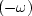to rotation in the other direction. To see why, suppose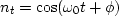and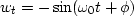.Then.The Fourier transform is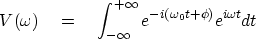(43)
The integrand oscillates and averages out to zero, except for the frequency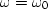.So the frequency function is a pulse at: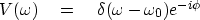(44)
Conversely, if wt were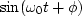,then the frequency function would be a pulse at,meaning that the wind velocity vector is rotating the other way.Next: Time-domain conjugate Up: CORRELATION AND SPECTRA Previous: Common signals
Stanford Exploration Project
10/21/1998